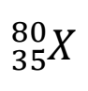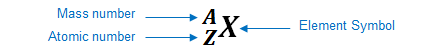# Problem: What element does “X” represent in the following symbol? a. Mercury (Hg)b. Chlorine (Cl)c. Rhodium (Rh)d. Scandium (Sc)e. Bromine (Br)

###### FREE Expert Solution

We’re being asked to determine to determine the element does “X” represent in the following symbol.An element can be represented as:You can determine the identity of the element using its atomic number:

Atomic number (Z):

88% (206 ratings)###### Problem Details

What element does “X” represent in the following symbol?a. Mercury (Hg)
b. Chlorine (Cl)
c. Rhodium (Rh)
d. Scandium (Sc)
e. Bromine (Br)